## Moment

Theth moment of a distribution about zerois defined by(1)

where(2), the Mean, is usually simply denoted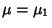. If the moment is instead taken about a point,(3)

The moments are most commonly taken about the Mean. These moments are denoted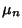and are defined by(4)

with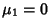. The moments about zero and about the Mean are related by(5)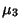(6)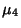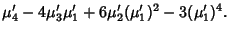(7)

The second moment about the Mean is equal to the Variance(8)

where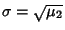is called the Standard Deviation.

The related Characteristic Function is defined by(9)

The moments may be simply computed using the Moment-Generating Function,(10)

A Distribution is not uniquely specified by its moments, although it is by its Characteristic Function.

See also Characteristic Function, Charlier's Check, Cumulant-Generating Function, Factorial Moment, Kurtosis, Mean, Moment-Generating Function, Skewness, Standard Deviation, Standardized Moment, Variance

References

Press, W. H.; Flannery, B. P.; Teukolsky, S. A.; and Vetterling, W. T. Moments of a Distribution: Mean, Variance, Skewness, and So Forth.'' §14.1 in Numerical Recipes in FORTRAN: The Art of Scientific Computing, 2nd ed. Cambridge, England: Cambridge University Press, pp. 604-609, 1992.